# 1. (60 points) Draw axial, shear, and bending moment diagrams for the frame shown below. Draw...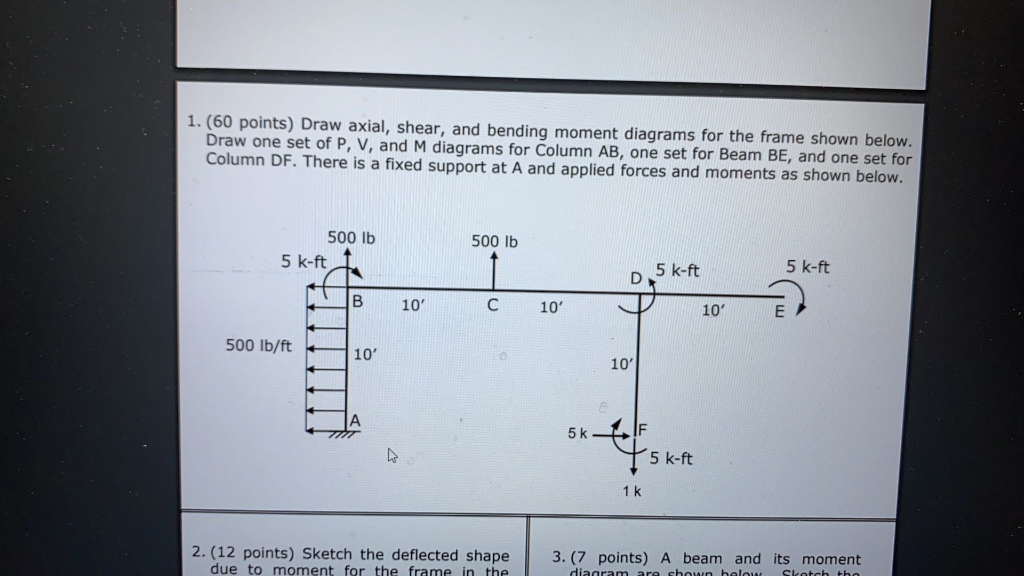1. (60 points) Draw axial, shear, and bending moment diagrams for the frame shown below. Draw one set of P, V, and M diagrams for Column AB, one set for Beam BE, and one set for Column DF. There is a fixed support at A and applied forces and moments as shown below. 500 lb 500 lb 5 k-ft 5 k-ft D 5 k-ft B 10 10' 10' E 500 lb/ft 10' 10' 5k s tiskt 5 k-ft 1k 2. (12 points) Sketch the deflected shape 3. (7 points) A beam and its moment dianramar ehun holaus Club due to moment for the frame in the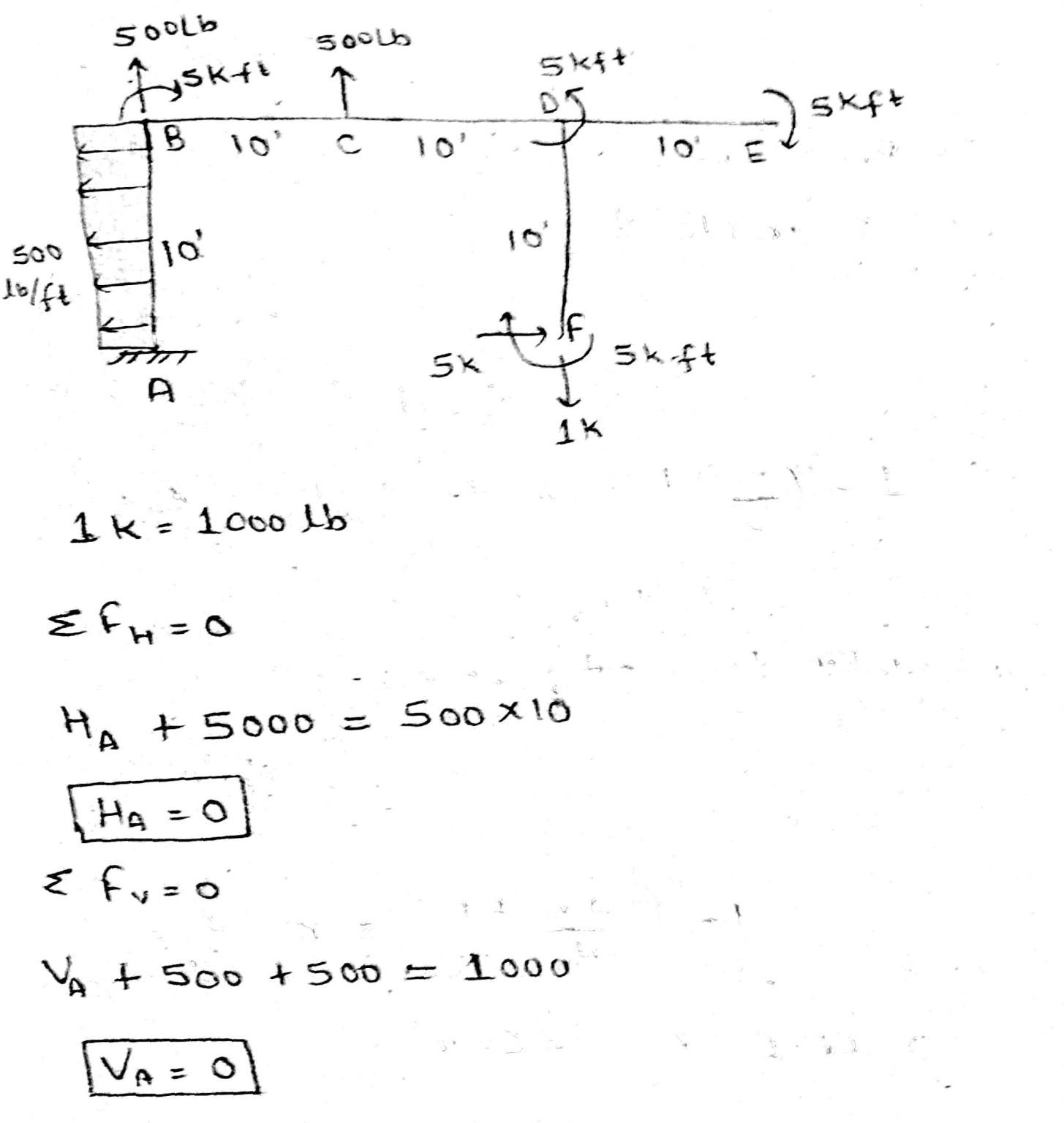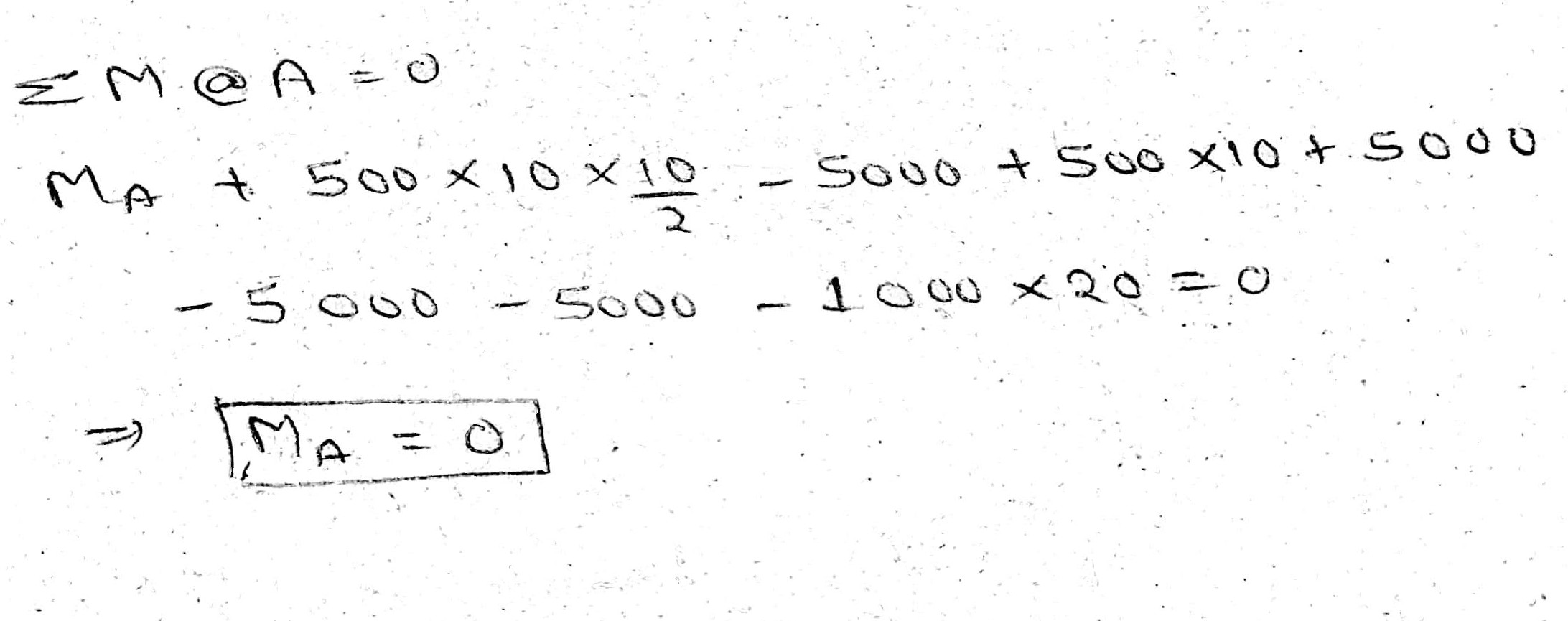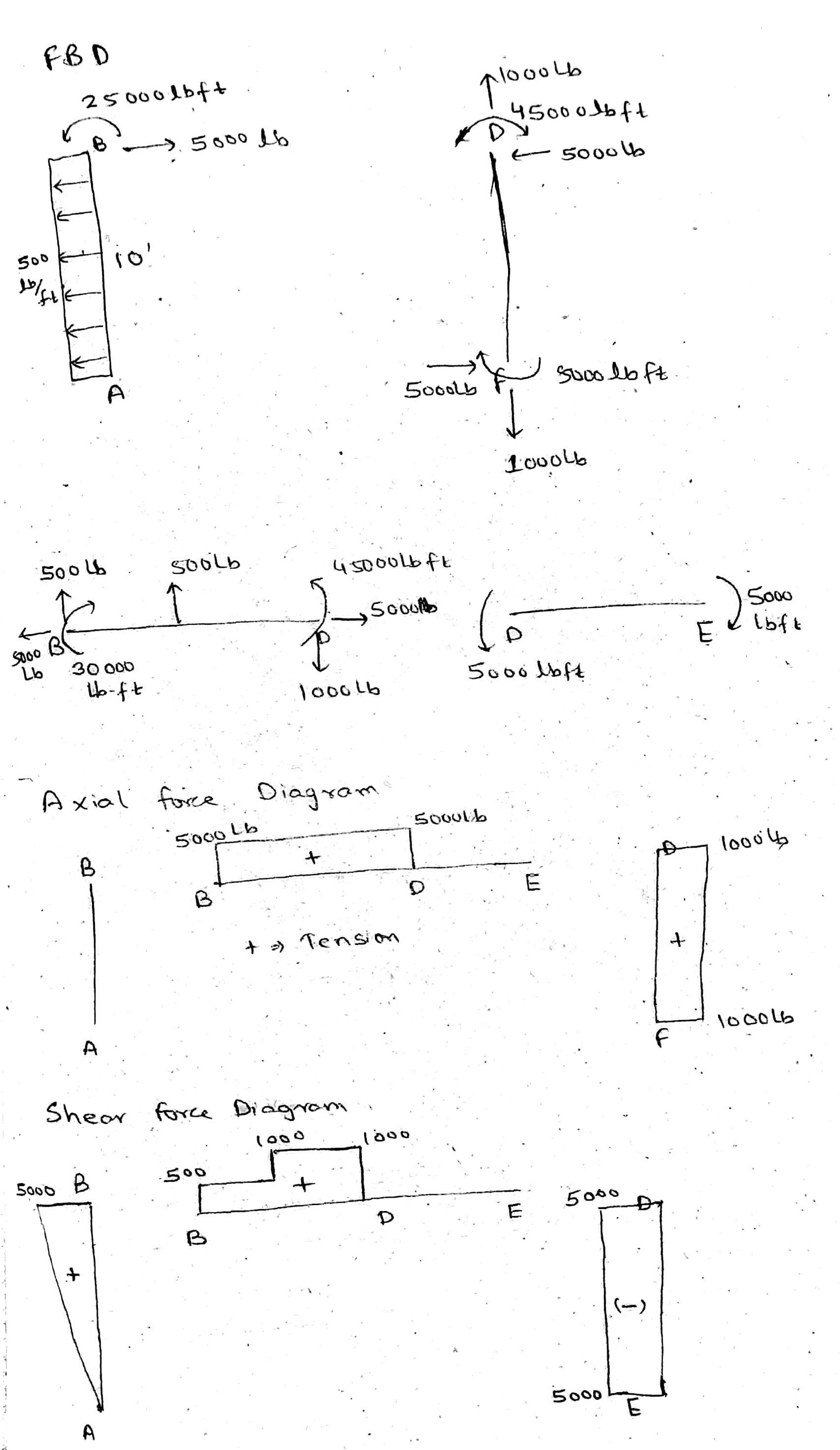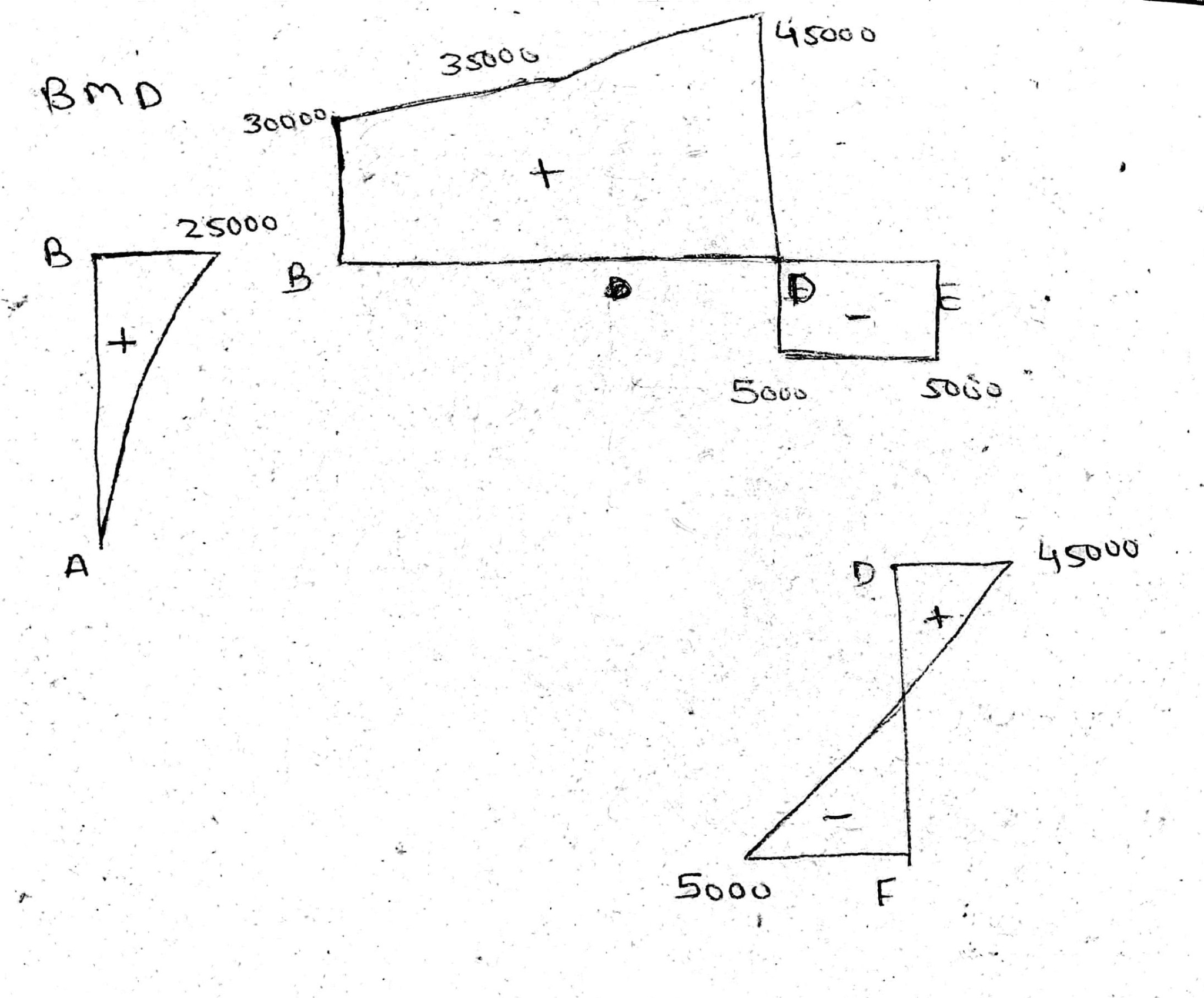#### Earn Coin

Coins can be redeemed for fabulous gifts.

Similar Homework Help Questions
• ### 4. After computing the reaction forces, draw the axial force N, shear force bending moment M...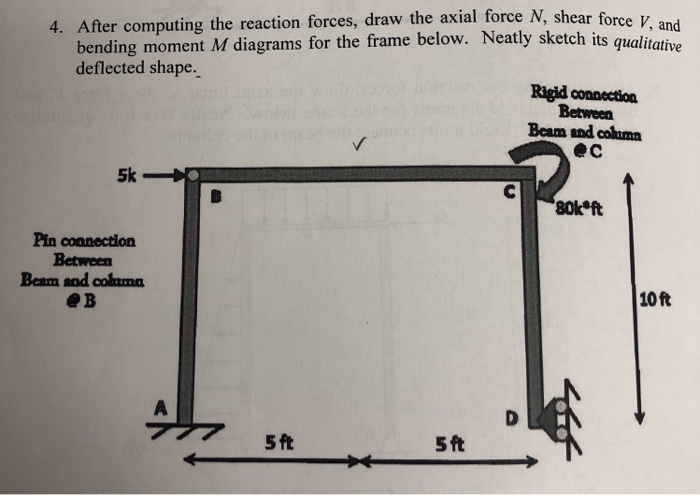4. After computing the reaction forces, draw the axial force N, shear force bending moment M diagrams for the frame below. Neatly sketch its quali deflected shape. Rigid connection Between Beam and colima 5k 80kft Pin connection Between Beam sod cohama eB 10 ft 5 ft 5 ft

• ### Problem 2 - Draw the shear and bending moment diagrams for the beam shown below. Clearly...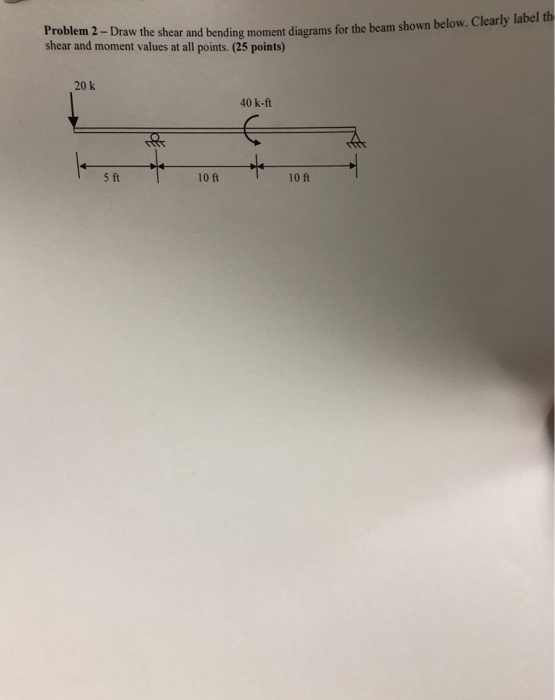Problem 2 - Draw the shear and bending moment diagrams for the beam shown below. Clearly label th shear and moment values at all points. (25 points) 20 k 40 k-ft 5 ft 10 ft 10 ft

• ### Draw the axial force, shear force, and bending moment diagrams for each member of the frame....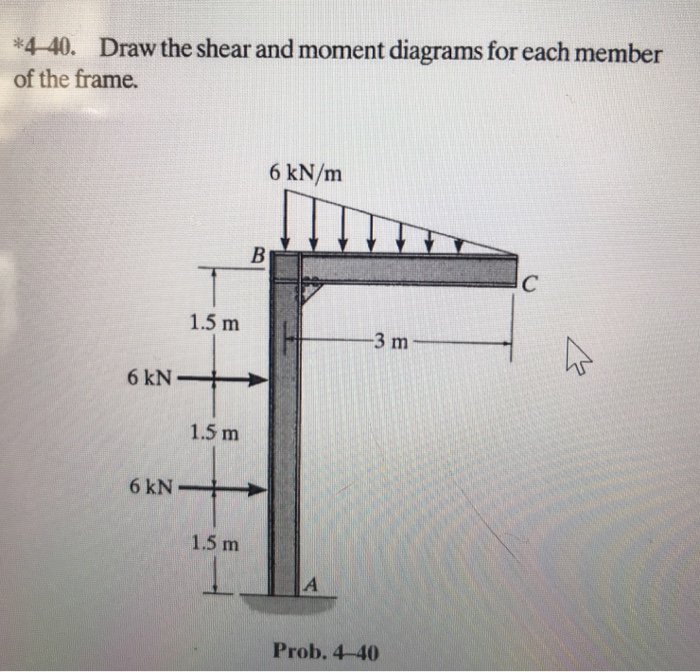Draw the axial force, shear force, and bending moment diagrams for each member of the frame. Additionally, determine the axial force, shear force, and bending moment considering the section located on member BC, 1m from C 4-40. Draw the shear and moment diagrams for each member of the frame. 6 kN/m 6 kN 1.5 m 1.5 m Prob. 4-40

• ### Draw the shear force and bending moment diagrams for the beam shown below. Clearly shovw the...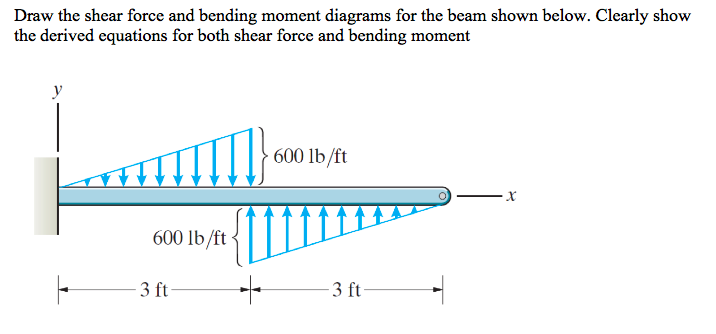Draw the shear force and bending moment diagrams for the beam shown below. Clearly shovw the derived equations for both shear force and bending moment 600 lb/ft 600 lb/ft 3 ft 3 ft

• ### For the following diagram, draw the shear and bending moment diagrams for the beam without formulating...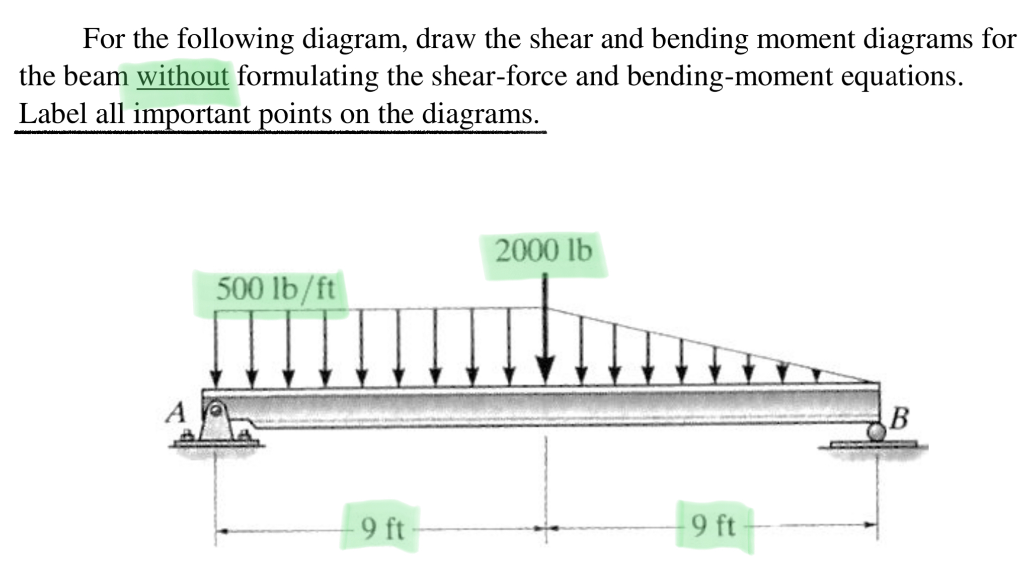For the following diagram, draw the shear and bending moment diagrams for the beam without formulating the shear-force and bending-moment equations. Label all important points on the diagrams. 2000 lb 500 lb/ft B 9 ft 9 ft

• ### Problem 7 Draw the complete axial force, shear force, and bending moment diagrams for each member...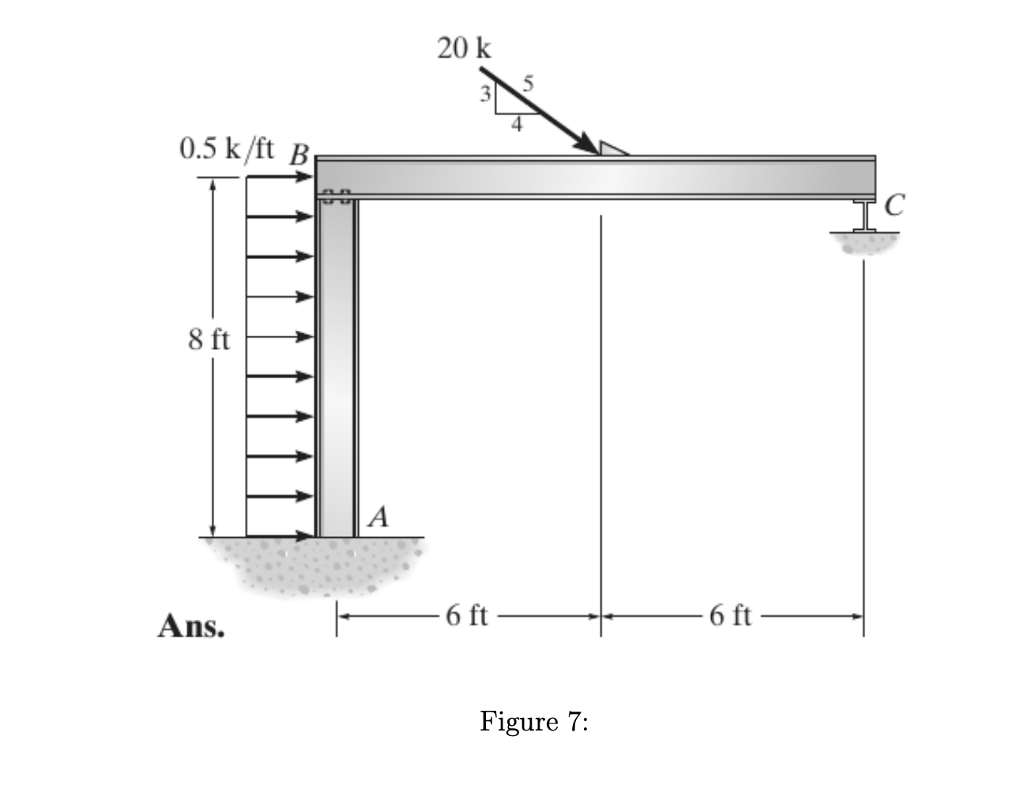Problem 7 Draw the complete axial force, shear force, and bending moment diagrams for each member of the frame shown in Figure 7. Assume A is fixed, the joint at B is a hinge, and support C is a roller. 20 k 5 3 0.5 k/ft B 8 ft 6 ft Ans. Figure 7:

• ### From the beam and loading shown, 9a) draw the shear-force and bending moment diagrams and (b)...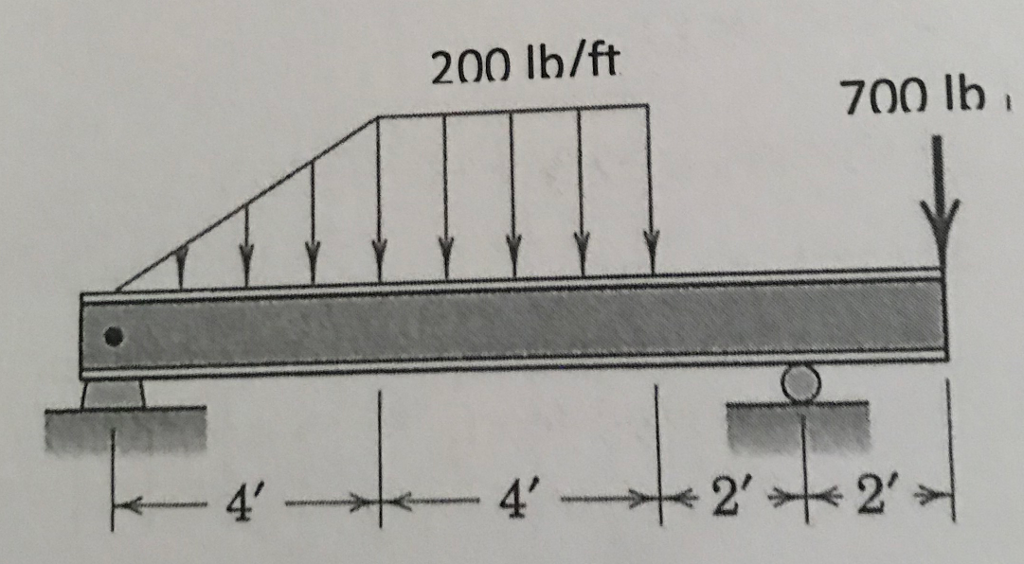From the beam and loading shown, 9a) draw the shear-force and bending moment diagrams and (b) determine the maximum absolute values of the shear and bending moment. please include free body diagrams for each set of equalibrium eqautions. 200 lb/ft 700 lb 4' 4'

• ### 3. Draw the shear and bending-moment diagrams for the beam and loading shown, and determine the...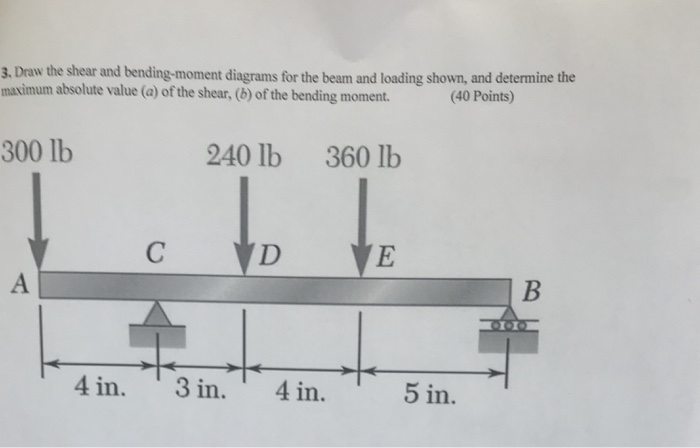3. Draw the shear and bending-moment diagrams for the beam and loading shown, and determine the maximum a bsolute value (a) of the shear, (b) of the bending moment. (40 Points) 300 lb 240 lb 360 lb CVDVE 4 in. 3 in. 4 in. 5 in.

• ### For the beam shown in the figure below Draw the shear and moment diagrams for this beam Calculate...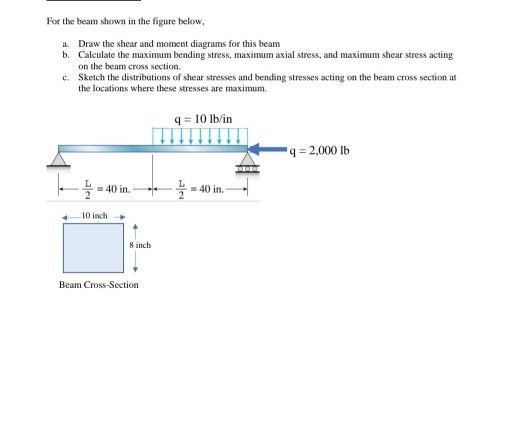For the beam shown in the figure below Draw the shear and moment diagrams for this beam Calculate the maximum bending stress, maximum axial stress, and maximum shear stress acting on the beam cross section Sketch the distributions of shear stresses and bending stresses acting on the beam cross section at the locations where these stresses are maximum. a. b. c. q 10 lb/in q 2,000 Ib 느-40 in ←_ 10 inch Beam Cross-Section For the beam shown in the...

• ### the axial force, shear force and bending moment diagramsFor the frame shown in Figure 10, Draw the axial force, shear force and bending moment diagrams for the frame, showing themagnitude of all peak values.Note: support A is a roller, support F is fixed, and there is an internal hinge at D.